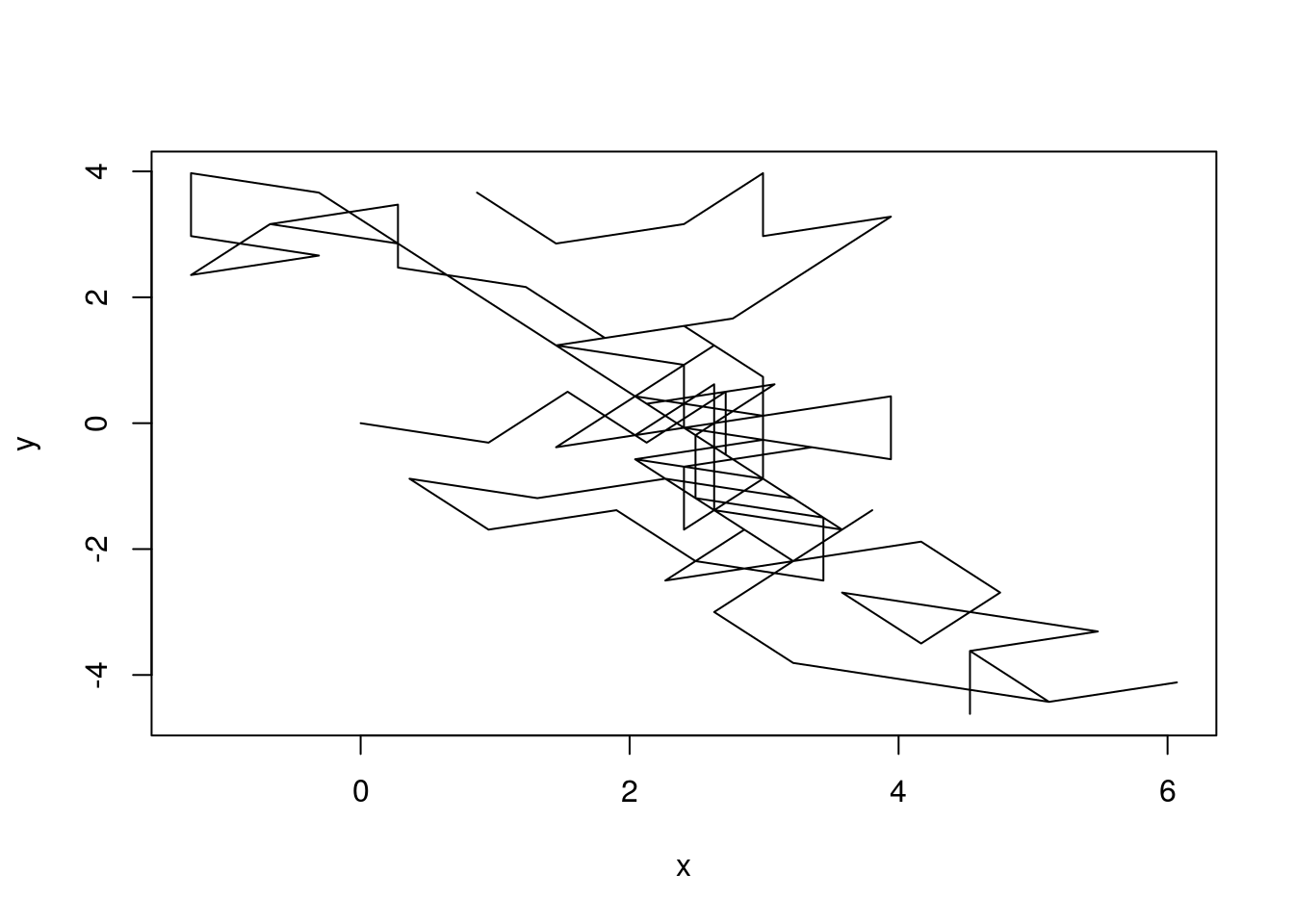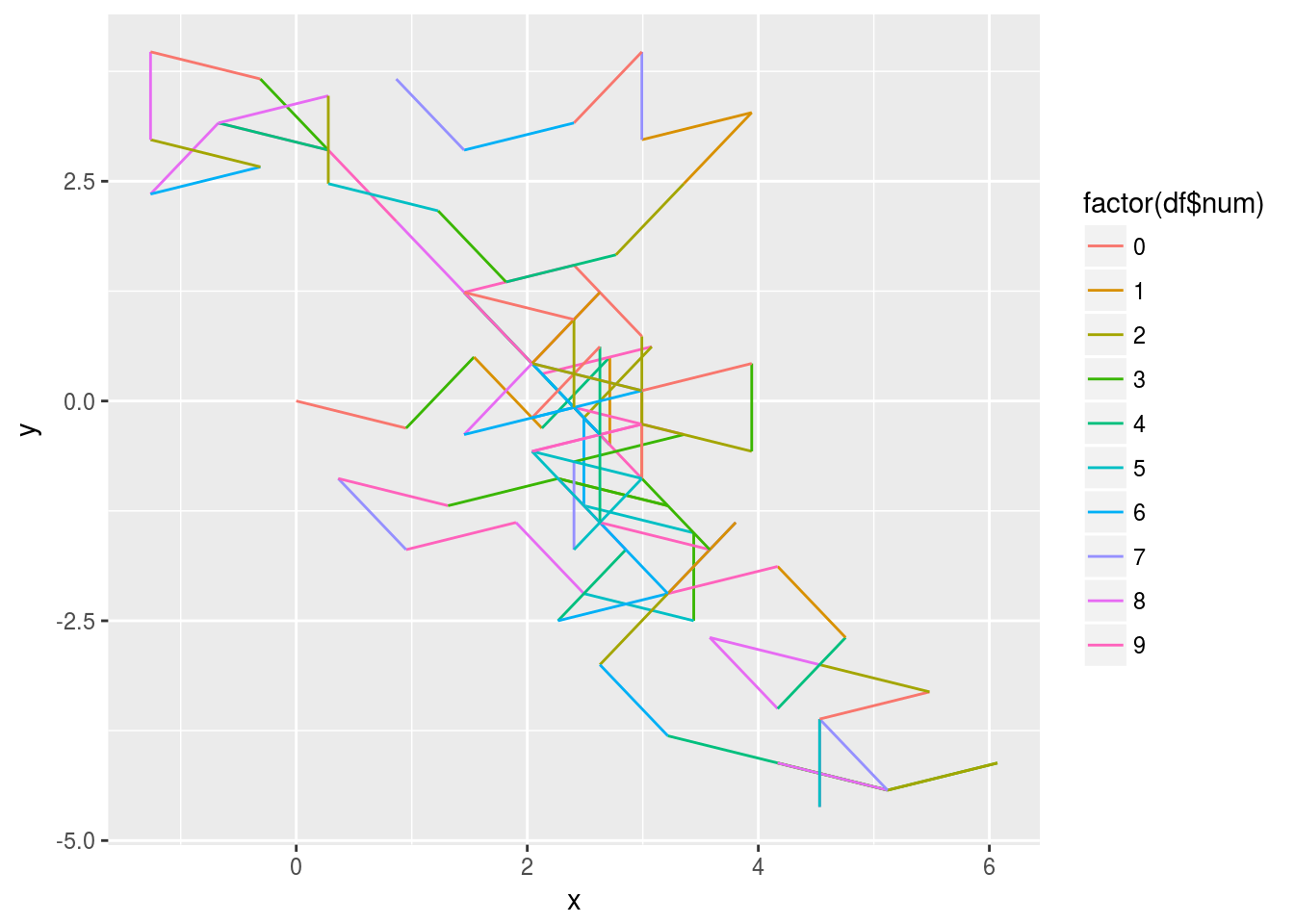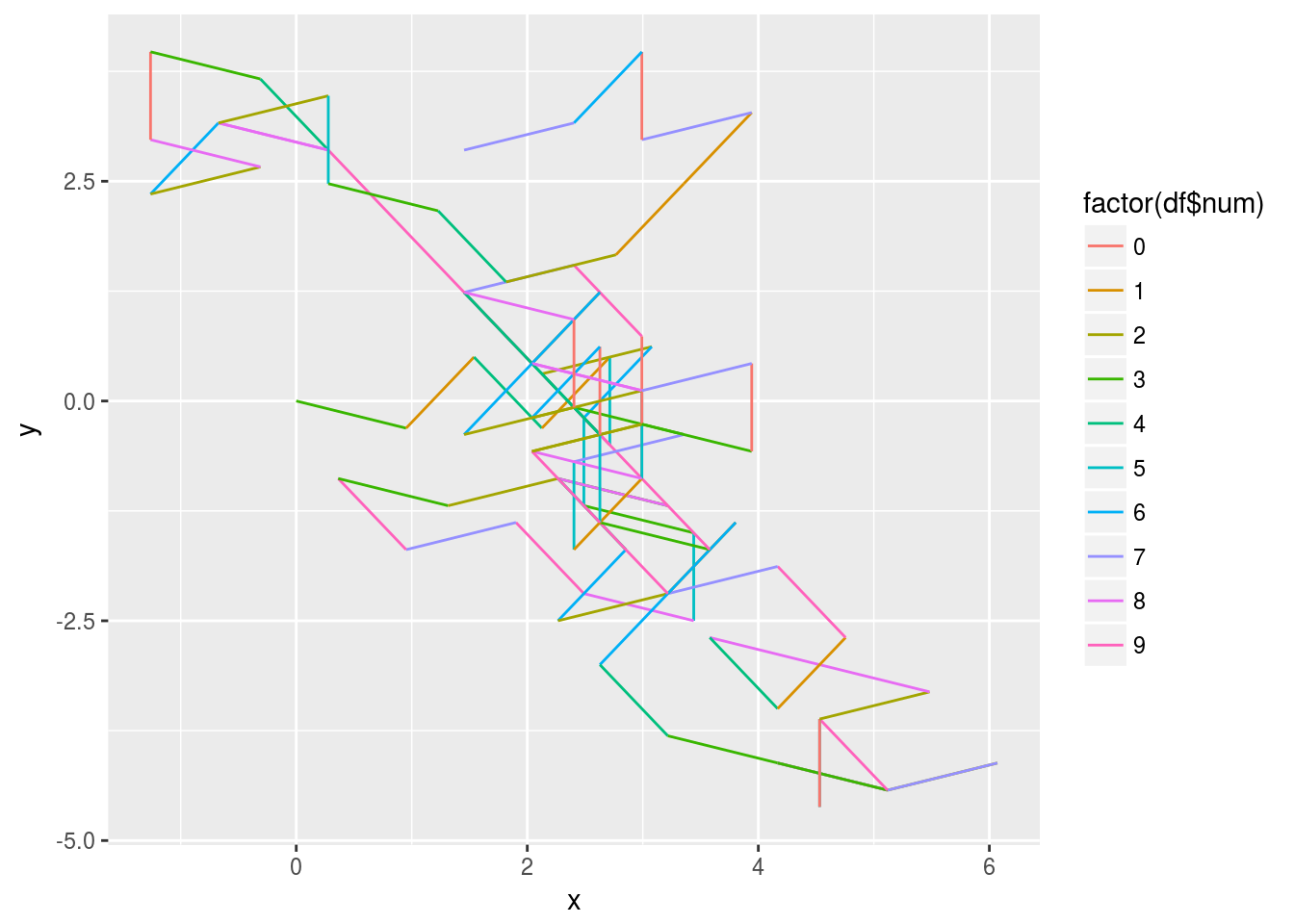# Euler problem 41

Project Euler is a pretty good way to exercise your programming muscles. I tend to think that the hardest part is usually the math.

So when I figure one of them out in minutes, I’m pretty happy about it.

```library(gtools) library(numbers) pandig <- function(n){ x <- permutations(n,n) x <- apply(x,1, function(x) paste(x, collapse="")) return(x) } y <- as.numeric(pandig(7)) max(y[isPrime(y)])```

The surprising thing is that largest pandigital prime does not begin with either 8 or 9.

# Practical project management

Project management is not easy. A handfull of practical hints from ~20 years of experience:

• Dont ask for more ressources. Ask for the ressources you were promised, but never got.
• Dont ask for an extension on deadlines. Ask that the ones you got in the first place are not changed.
• Never expect anyone to have heard of the iron triangle of project management.
Even if they actually have heard of it before.
• Never expect anyone to have any understanding of what the project is about.
Even if they are in charge of managing it.
• When, at the beginning of a project, estimations of the necessary ressources are deemed irrelevant by the steering commitee, buy antacids. You will get an ulcer.
• When someone is given a budget – never expect them to stay within it.

# Star Trek in stardate order

By popular demand: Star Trek in stardate order. Discovery is not in the list. I think we still have to figure out if it is a heretical series, or if it fits into the correct, and only true timeline.

# Clifford

I have a strange affinity for attractors, and has had it since graduating highschool, where I got a top grade in the final mathematics examination. That was under the old grading system. And the grade (13) was given to only two students in my high school that year. The examination centered on strange attractors. I’ve have not spend much time on it lateer. But there is a weird beauty in them.

Recently I discovered Clifford Attractors. Take a look at this page for some very nice examples. They look stunning, and are simple to handle. Lets play with them!

Clifford Attractors are defined by iteratively making these calculations: xn+1 <- sin(ayn) + ccos(axn) yn+1 <- sin(bxn) + dcos(b*yn)

Choose a, b, c and d between -2 and 2. Calculate x,y for n=0 to n=10.000.000 and plot them. It looks cool!

Lets begin by defining a function that takes four variables and a number of points, and calculate the points:

``````calcTrace <- function(a,b,c,d,numint){
x <- y <- rep(NULL, numint) # initializing the vectors
x <- 0 # set the first point to (0,0)
y <- 0
for (i in 2:numint){    # calculate the following points
x[i] <- sin(a*y[(i-1)]) + c*cos(a*x[(i-1)])
y[i] <- sin(b*x[(i-1)]) + d*cos(b*y[(i-1)])
}
df <- data.frame(x=x,y=y)
return(df)
}``````

Lets also define some parameters. I would prefer to choose the parameters at random. But there are a surprising number of instances where this lead to nothing. The formulas converges very quickly on just a few values, and I end up with a simple dot on the plot. I guess that is to be expected – but not what I am looking for. I want beautiful images!

``````a <- -1.8
b <- -1.9
c <- -1.7
d <-  1.9

points <- calcTrace(a,b,c,d,10000000)``````

That takes some time – I’ll get back to that. Lets plot it. I remove almost anything from the ggplot theme, and insert the parameters in the plot.

``````library(ggplot2)
opt = theme(legend.position  = "none",
panel.background = element_rect(fill="white"),
axis.ticks       = element_blank(),
panel.grid       = element_blank(),
axis.title       = element_blank(),
axis.text        = element_blank(),
plot.title       = element_text(size=9, face="italic", hjust = 0.5)
)

p <- ggplot(points, aes(x, y)) + geom_point(color="black", shape=46, alpha=.01) +
opt +
ggtitle(paste("a = ",a , ", b = ", b, "\n c = ", c, " d = ",  d))
print(p)``````All right. It takes some time to do the calculations. There are ways to speed that up.

One way is to compile the function.

``````library(compiler)
compiled <- cmpfun(calcTrace)``````

According to the compiler packages, that should make the function faster. Lets test it:

``````library(microbenchmark)
test <- microbenchmark(
calcTrace(a,b,c,d,10000000),
compiled(a,b,c,d,10000000) , times=3
)
print(test)``````

I am not impressed. This result might be caused by the fact that I am running Paperclips http://www.decisionproblem.com/paperclips in the background (almost ready to release the hypnodrones!) on a not particularly powerfull laptop.

What else could be done? I’m tinkering with this to hone my R-skills. But here might be a situation where it would be better to do it in another language.

The library Rcpp allows me to add C++ code. Lets try that:

``````library(Rcpp)
cppFunction('DataFrame cppTrace(double a, double b, double c, double d, int numint) {
// create the columns
NumericVector x(numint);
NumericVector y(numint);
x=0;
y=0;
for(int i = 1; i < numint; ++i) {
x[i] = sin(a*y[i-1])+c*cos(a*x[i-1]);
y[i] = sin(b*x[i-1])+d*cos(b*y[i-1]);
}
// return a new data frame
return DataFrame::create(_["x"]= x, _["y"]= y);
}
')``````

Lets see how quick that version is:

``library(microbenchmark)``
``## Loading required package: microbenchmarkCore``
``````test2 <- microbenchmark(
cppTrace(a,b,c,d,10000000),
times=3
)
print(test2)``````
``````## Unit: seconds
##                         expr     min       lq     mean   median       uq
##  cppTrace(a, b, c, d, 1e+07) 2.11005 2.122835 2.154553 2.135619 2.176804
##       max neval
##  2.217989     3``````

That was fast!

The plot still takes an awfull lot of time. I have not found a way to do anything about that.

Lets make a new plot, with different parameters.

``````a <-  1.7
b <-  1.7
c <-  0.7
d <-  1.3
points <- cppTrace(a,b,c,d,10000000)
q <- ggplot(points, aes(x, y)) + geom_point(color="orange", shape=46, alpha=.01) +
opt +
ggtitle(paste("a = ",a , ", b = ", b, "\n c = ", c, " d = ",  d))
print(q)``````Nice. Lets make one in green as well:

``````a <-  -1.6
b <-  1.2
c <-  0.1
d <-  -1.2
points <- cppTrace(a,b,c,d,10000000)
r <- ggplot(points, aes(x, y)) + geom_point(color="green", shape=46, alpha=.01) +
opt +
ggtitle(paste("a = ",a , ", b = ", b, "\n c = ", c, " d = ",  d))
print(r)``````# How do you gauge a place to work

I’ve recently been involved in the recruitment of a mid-level manager in an organization I’m involved with. That got me thinking (always a good way to prepare for anything):

What questions are reasonable to ask? There are rules of course, but what kind of questions is it OK for the prospective new employee to ask.

There are all the professional questions. But you should also be interested in what kind of a workplace you are potentially entering.

I think a good question is: What kind of employee benefits do you offer? And why those?

The added question is important. I am not interested in knowing that you offer all the fruit I can eat. That is not what will decide if I want to take the job.

But it does make a difference if company A offers free coffee. And company B offers me all the water and electricity I will need to brew coffee on the brewer I have to bring myself, using coffee grounds that I will have to buy myself.

And it would be interesting to hear a manager reflect on why they offer free yoga, coffee or whatever.

I think it says a lot about how employees are viewed in a company.

# Merging pdf-files

You may need to merge several pdf-files into one.

There are several tools. Some of them you have to pay for.

If you are in a linux environment, try this:

gs -dBATCH -dNOPAUSE -q -sDEVICE=pdfwrite -sOutputFile=final.pdf filea.pdf fileb.pdf

continue with filec.pdf etc.

Some say it is slow.

Others say, yes it is slow, but it produces smaller final files, and are able to handle “difficult pdfs”.

I say it is nice that it can be done from the command line.

# Visualizing Pi

We celebrate Pi-day (3/14) every year at the library. And though it is december, and there is months to the big day, it is not too early to begin planning. This is something we do in the incredibly amounts of time we have left over when all the other tasks that also only take a tiny portion of the day are done. In other words, this is something we plan in our weekly lunchbreak.

Usually Henrik delivers a small talk about Pi. We eat some Pie, and there is much rejoicing.

But we would like to show something else. A cool visualization perhaps. There are several out there, but it is no fun just to show cool stuff. Its much cooler to understand how it is done.

One of the visualizaions we found on the net is this. https://www.visualcinnamon.com/portfolio/the-art-in-pi It is made by the extremely talented Nadieh Bremer. Take a look at her page. She does a lot of other cool stuff!

So. How to replicate this?

The idea is to take pi. Draw a line segment from 0,0 in the plane to a new point, determined by the first digit. Then we draw another segment from that point, to a newer point, determined by the second digit in Pi. Continue ad nauseam, or until your computer catches fire. Add colours, and make it look cool.

First item is to get pi to a million decimal places. Read it in, and make a vector containing all the digits. I’ll begin with a smaller string.

``testnumvec <- "3.1415926535897932384626433832795028841971693993751058209749445923078164062862089986280348253421170679"``

Not quite pi. But I can’t be bothered figuring out how many digits of pi I should use in order to get all the digits from 0 to 9. I want that to make sure that whatever I do, works on all the digits.

Next up is getting rid of the period.

``numvec <- gsub("[[:punct:]]", "", testnumvec)``

In Denmark we would use a “,” instead of “.” This removes all punctuation.

Now we convert it to a vector with individual digits. And cast them as integers rather than characters.

``numvec <- as.integer(unlist(strsplit(numvec,"")))``

We begin a (0,0). The next point is determined by the first digit i pi – 3. The number 3 should determine witch direction, angle, we should move. The new x-value will be cos(f(3)), where f(3) is some function, that converts 3 to the correct angle. That should be some fraction of pi. The new y-value is similarly determined by sin(f(3)).

What should f(x) be? Imagine a decimal clock, with the ten digits 0 to 9 positioned around the circle. In the original 0 is at top, at 12 o’clock. 5 is at 6 o’clock. And the numbers follow the circle clockwise. In the unity circle 0 is positioned at 1/2pi. 5 at 1 1/2 pi. So f(0)=1/2pi, f(5)=1 1/2pi.

This formula gives us the result we need: 5/2pi – z/5pi.

z is the digit in pi. For all of them, we can calculate the steps we need in this way:

``````x <- cos(5/2*pi - numvec/5*pi)
y <- sin(5/2*pi - numvec/5*pi)``````

So. Beginning at (x0,y0), we calculate the trig functions, add it to (x0,y0) and get (x1,y1). When we continue to do that, we notice, that xi is the cumulative sum of all the calculated x-values. If we calculate the trig functions for all of the digits in pi, and then calculate the cumulative sum, we get all the x-values. Similarly with all the y-values.

Thats neat, and thanks to Henrik, who pointed this out. We get the cumulative sums easily: And we can then get the cumulative sums with:

``````x <- cumsum(x)
y <- cumsum(y)``````

Thats it! There is just a little detail. The first value in x,y is the first step we need to take – or the second point if you will. We will need to add a 0 at the start:

``````x <- c(0,x)
y <- c(0,y)``````

Thats it! I should now be able to plot it. Lets just do a quick test:

``plot(x,y, type="l")``That looks almost like what we need. The most notable difference is that Nadieh only plots the decimals. I include the “3” as well. We are going to test this, and it is cumbersome to do all the preceding steps. Let’s begin with defining the generation of points as a function, that takes a string. In just a little while I’ll invoke the magick of ggplot. So it would be convenient that the result is a dataframe:

``````piPoints <- function(piString){
numbers <- gsub("[[:punct:]]", "", piString)
numbers <- as.integer(unlist(strsplit(numbers,"")))
x <- cos(5/2*pi - numbers/5*pi)
y <- sin(5/2*pi - numbers/5*pi)
x <- cumsum(x)
y <- cumsum(y)
x <- c(0,x)
y <- c(0,y)
df <- data.frame(as.integer(c(0,numbers)),x,y)
colnames(df) <- c("num", "x", "y")
return(df)
}``````

All right. We now have a dataframe with three columns. The number, and the x,y coordinates. We can plot that with ggplot:

``````df <- piPoints(testnumvec)
library(ggplot2)

ggplot(df, aes(x=x,y=y,group="1"))+
geom_path(aes(colour=factor(df\$num)))``````Weird. Something is wrong. Look at the second linesegment. It goes up and to the right, just as it should, since this segment represents a “1”. But the color? It is green. It should be sorta orangy. The reason is that ggplot looks at the number, and by implication the color, at the origin of the linesegment. Not at the end. How to change that? What I really need to do, is shifting the number column one place relative to the x,y pairs. Simply done – remove the 0 at the beginning of the num-vector in the function. And the final digits in the x and y vectors. I really don’t like it, as it increases the size of the dataframe – but I add an id-column as well. Just a sequential number, to control the colouring:

``````piPoints <- function(piString){
numbers <- gsub("[[:punct:]]", "", piString)
numbers <- as.integer(unlist(strsplit(numbers,"")))
x <- cos(5/2*pi - numbers/5*pi)
y <- sin(5/2*pi - numbers/5*pi)
x <- cumsum(x)
y <- cumsum(y)
x <- c(0,x)
y <- c(0,y)
id <- 1:(length(y)-1)
df <- data.frame(as.integer(c(numbers)),x[-length(x)],y[-length(y)], id)
colnames(df) <- c("num", "x", "y", "id")
return(df)
}``````

Lets take another look:

``````df <- piPoints(testnumvec)
library(ggplot2)

ggplot(df, aes(x=x,y=y,group="1"))+
geom_path(aes(colour=factor(df\$num)))``````Now we’re there. Two steps are missing: Adding more digits – I would like to get to one million digits. And getting it to look nice.

Lets try getting to the million. I don’t need to calculate pi to a million decimals. I can just download it.

``````library(readr)